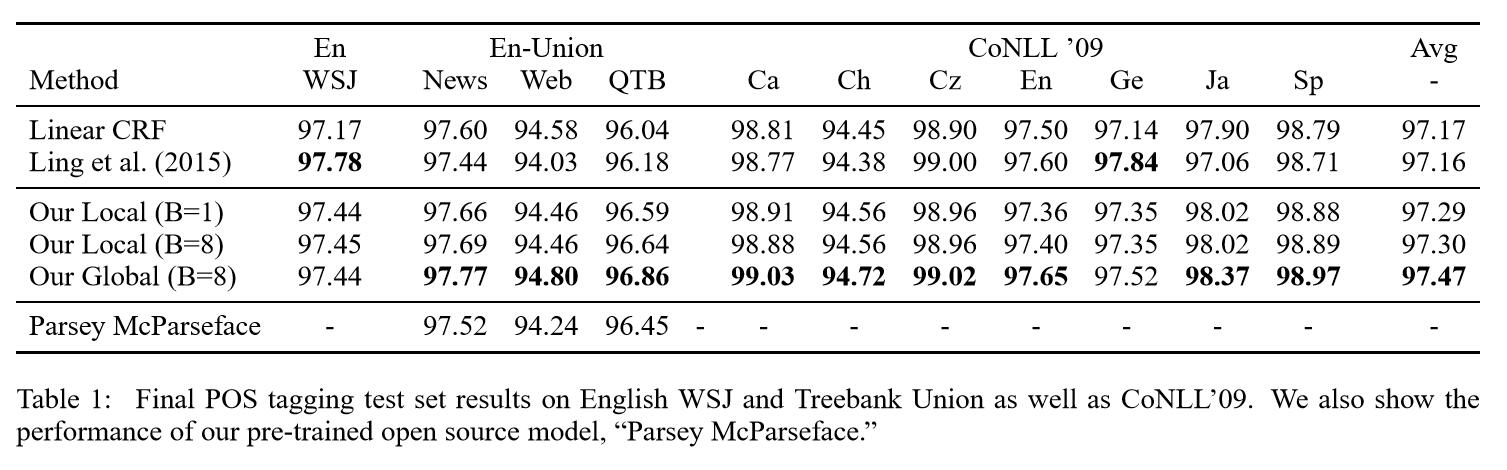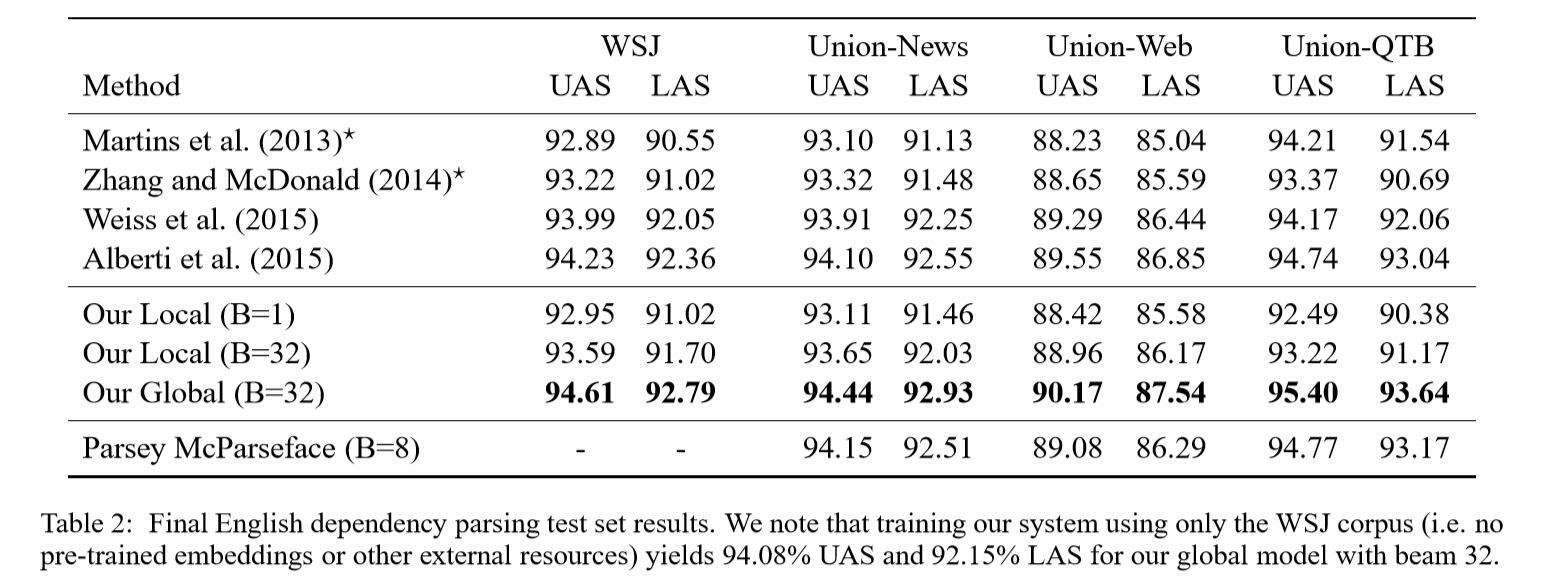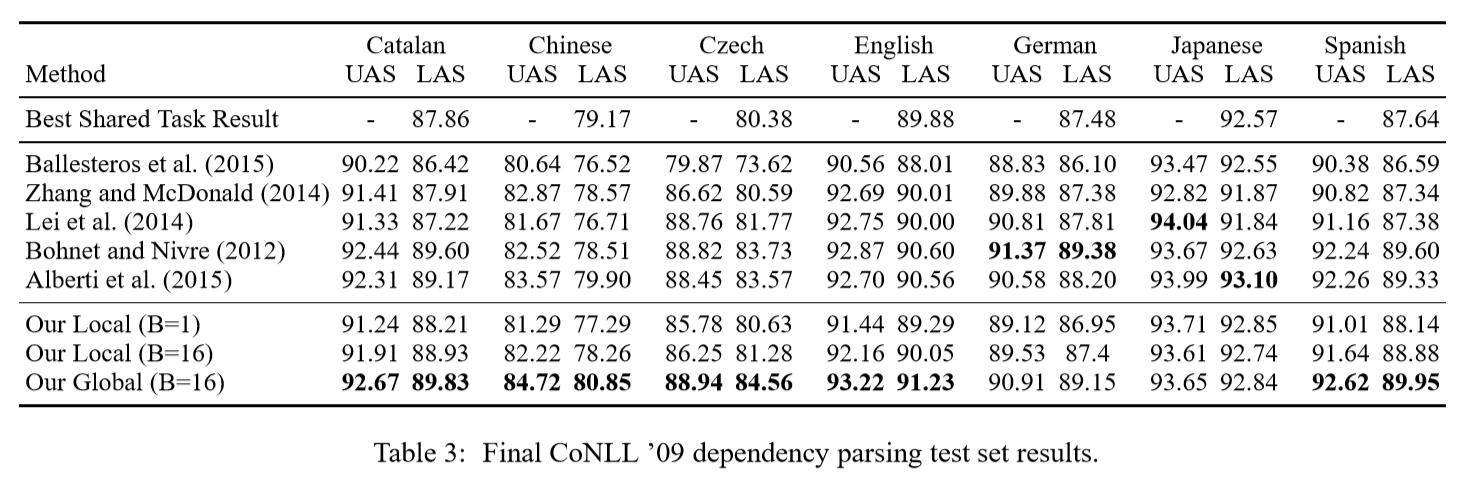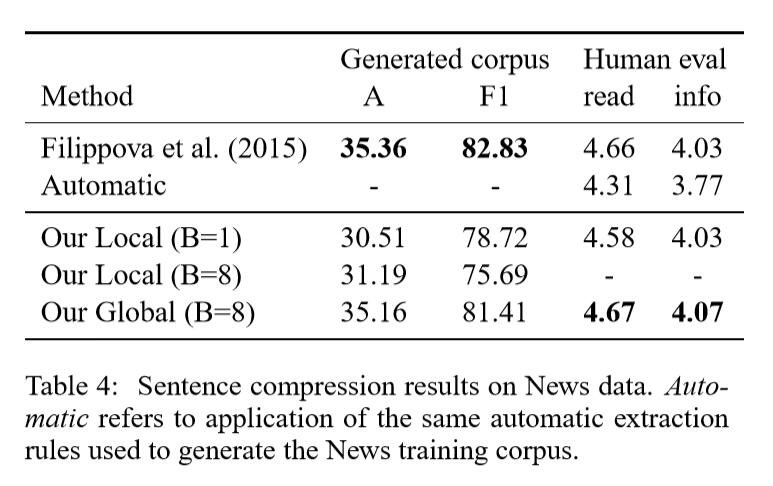SyntaxNet :Globally Normalized Transition-Based Neural Networks
Daniel Andor, Chris Alberti, David Weiss, Aliaksei Severyn et.al
Google Inc

# Introduction

NN在NLP领域应用广泛，LSTM在POS,语法解析，语义角色标注应用广泛。一个观点是因为循环神经网络才得到这样的效果

# 2 Model

## 2.1 Transition system

• 状态集合$S(x)$
• 特定的开始符号 $S^{\dagger} \in S(x)$
• 允许的决策集合 $A(s,x)$, 所有的$s \in S(x)$
• 转移函数 $t(s,d,x)$, 对任一决策$d \in A(s,x)$返回一个新的状态$s^\prime$

$$\rho(s,d;\theta)=\phi(s;\theta^{(l)}) \cdot \theta^{(d)}.$$
$\theta^{(l)}$ 是神经网络出去最后一层的所有参数。$\theta^{(d)}$ 是对决策$d$的最后一层参数。 $\phi(s;\theta^{(l)})$ 表示神经网络在参数 $\theta^{(l)}$ 计算得到的状态 $s$。注意，在参数 $\theta^{(d)}$下得分是线性的。我们之后会解释 softmax-style normalization 是如何应用在global和local级的。

## 2.2 Global vs. Local Normalization

$$p(d_j | d_{1:j-1;\theta}) = \frac{\exp \rho(d_{1:j-1}, d_j; \theta)}{Z_L(d_{1:j-1}; \theta)} \tag{1}$$

$$Z_L(d_{1:j-1}; \theta) = \sum_{d^\prime \in A(d_{1:j-1})} \exp \rho(d_{1:j-1},d^\prime; \theta)$$

$$p_L(d^{1:n} = \prod_{j=1}^n p(d_j | d_{1:j-1; \theta})\ =\frac{\exp \sum_{j=1}^n \rho(d_{1:j-1, d_j; \theta})}{\prod_{n=1}^{n}Z_L(d_{1:j-1}; \theta)}. \tag{2}$$

$$p_G(d_{1:n}) = \frac{\exp \sum_{j=1}^n \rho(d_{1:j-1}, d_j; \theta)}{Z_G(\theta)} \tag{3}$$

$$Z_G(\theta) = \sum_{d_{1:n}^\prime \in D_n} \exp \sum_{j=1}^n \rho(d_{1:j-1}^\prime, d_j^\prime; \theta)$$
$D_n$是所有的长度为n的有效决策序列集合， $Z_G(\theta)$是global normalization 项。现在推断问题成为找到：
$$\text{argmax}_{d_{1:n} \in D_n} = \text{argmax}_{d_{1:n}\in D_n} \sum_{j=1}^n \rho(d_{1:j-1}, d_j; \theta).$$

## 2.3 Training

$$L_{local}(d_{1:n}^ ;\theta) = -\ln p_L(d_{1:n}^;\theta) = \ -\sum_{j=1}^n \rho(d_{1:n}^, d_j^; \theta) + \sum_{j=1}^n\ln Z_L(d_{1:n}^; \theta) \tag{4}$$

$$L_{global}(d_{1:n}^;\theta) = -\ln p_G(d_{1:n}^;\theta) = \ -\sum_{j=1}^n \rho(d_{1:n}^, d_j^; \theta) + \sum_{j=1}^n\ln Z_G(d_{1:n}^*; \theta) \tag{5}$$

locally normalized 的一个显著优势是公式4中 $Z_L$及其导数计算快速有效。而Eq.5中 $Z_G$ 项在很多情况下是难以处理的。

$$L_{global-beam}(d_{1:n}^:\theta) = \ -\sum_{i=1}^j \rho(d_{1:i-1}^ ;\theta) + \ln \sum_{d_{1:j} \in \mit{B_j} } \exp \sum_{i=1}^j \rho(d_{1:n}^\prime, d_i^\prime; \theta) . \tag{6}$$

# 3 The Label Bias Problem

Global Models can be Strictly More Expressive than Local Models

$$p_L(d_{1:n} | x_{1:n}) = \prod_{i=1}^n p_L(d_i|d_{1:i-1}, x_{1:i}) \ = \frac{\exp \sum_{i=1}^n \rho(d_{1:i-1}, d_i, x_{1:i})}{\prod_{i=1}^n Z_L(d_{1:i-1}, x_{1:i})}$$

$$p_G(d_{1:n} | x_{1:n}) = \frac{\exp \sum_{i=1}^n \rho(d_{1:i-1}, d_i, x_{1:i}) }{Z_G(x_{1:n})}$$

# 4 Experiments

## 4.1 POS

• the English Wall Street Journal (WSJ) part of the Penn Treebank
• the English “Treebank Union” multi-domain corpus containing data from the OntoNotes corpus version 5
• the CoNLL ’09 multi-lingual shared task

Model Conﬁguration
Result## 4.2 依赖解析

Model Conﬁguration

Result## 4.3 语句压缩

Filippova(2015)数据集。
Model Conﬁguration

Result